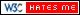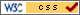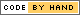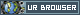# Autonomous Sniffer Robot

## Introduction

We build an robot that detects WLAN flood attacks and navigates itself towards the intruding device's locality with obstacle evasion functionality. Click here to see our video

## Objective

- The system must be able to parse captured frames and filter out malicious ones in real time.

- The robot can find out the direction in which the intruder resides, with the help of signal strength data acquired from the rotational antenna.

- The robot will be able to evade simple obstacles like boxes or wall, using the ultrasonic sensor.

## Design

### 1. Capture of 802.11 frames

Firstly, we switch the wireless adapter into monitor mode. Then we use a Python library called "scapy" to control that wireless adapter and make it capture 802.11 frames regardless of receiver's MAC adress. After capture, we parse all the frames, find out the malicious ones and read signal strength from the headers of those frames. All above is done in real time.

### 2. Rotational Antenna

We install a directional antenna to the wireless adapter. The wireless adapter is mounted on a standard servo that can rotate from -90 to +90 degrees. We partition those 180 degrees into 8 pieces, which means 9 directions. The servo propels the antenna to rotate, in each direction, the antenna stays for half second. During that period of time, the system calculates the average signal strength of the frames captured in that direction.

The robot is propelled by two servos driving two wheels respectively. At first the robot stands still and wait the antenna to scan all nine directions. Then the robot picks up the direction with maximum average signal strength, turns to that direction, and starting marching forward. The antenna keeps scanning, replacing old data with new one. When a new maximum direction is found, the robot will turn to that direction and moves forward. As the robot is appoaching the intruding device, the average signal strength will grow larger and larger; when it reaches the threshold we choose, the robot will stop there and sound the siren sound, indicating the intruders location.

### 4. Obstacle Evation

We mount a ultrasonic range finder on the wood leg of the vehicle. The sensor detects the distance ahead when it receives a trigger signal. We constantly trigger the sensor, at a frequency of 1Hz, to get up-to-date distance information. When the distance ahead is less than 30 centimeters, an obstacle is detected, the robot will take a evasive maneuver by turning 90 degrees and then moving forward for 2 seconds, where left or right is determined by the bigger one of the average signal strength of left side and right side. Then the robot stops and its antenna continues rotating and scanning.

## Testing

For the testing we used two kinds of environments. The first one is in the lab. We put the intruder on the table and the robot on the floor. With no obstacles in between but walls and table legs will still be around to be the boundary. The robot will be positioned with an angle to the intruder(even backwards). The robot will be started and autonomous find the intruder direction and move toward it.

The second environment is in the hall way where there are walls restricting the movements of the robot. The intruder will be positioned on a chair or on the floor. The robot will be positioned about 20-30 feet from the intruder and the robot will face the wall with an angle. During the test, we will use random obstacle(a foot) to block the robot. However, we will not put obstacles directly in between the intruder and the robot since when the robot is avoiding obstacles, it will try to find where the stronger singal is. So if obstacles are put in between the robot and the intruder, the robot will be stuck unless the obstacle could block signals.

## Overview of the robot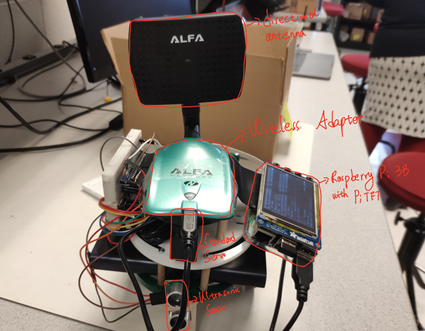## Rear View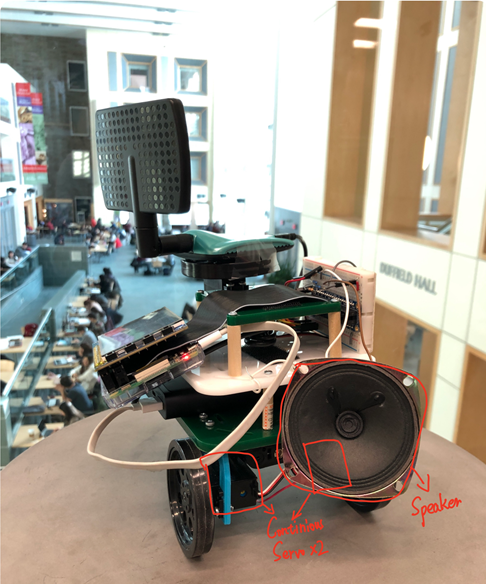## Problems

1. The wireless adapter on the Pi's board does not have monitor mode. So we have to mount another adapter to the Pi.

2. In this project we have several GPIO devices working at the same time, so we have to implement multi-thread. We discover that the ultrasonic sensor works badly in a thread but it works well in an independent process. We have too many threads that outputs to GPIO pins and we conjecture that they may interfere with each other.

3.  With only one ultrasonic sensor, we could avoid one simple obstacle(pillar or box) at a time. It could also avoid bumping into walls in the hallway. However, it couldn't handle complex terrain or obstacles. More sensors are required for this situation including ultrasonic sensor or even cameras with computer vision.

## Results

The result is acceptable, the sniffer robot can eventually navigate itself to the location of the intruding device. However, if the signal has reflected multiple times before it reaches the robot, the robot may strictly follow the trace of the signal and march in a zic-zac fashion.

## Conclusion

Our project produced an autonomous sniffer robot which can detect WLAN flood attack sources and move towards the attacker/intruder. When it receives a singal above threshold value, it will stop to inform the user that the intruder is within a small range. It's efficient in detecting flood attacks and it can catch the intruder in one minute. Most of the WLAN flood attacks happen in less than 5 minutes and it's hard to find them on the software level. Our project sloved this problem utilizing the Raspberry Pi platform. What's more, our project involves multithreading and multiprocessing to make full use of the Raspberry Pi A53 cores.

## Future

This project could be enhanced by added more elegant obstacle avoiding or detouring algorithms. Also, the robot can just do triangulation location instead of moving towards the signal source.

## Budget

 Parts Cost per item quantity Sum Alfa directional antenna \$10.99 1 \$10.99 Alfa wireless adapter \$29.99 1 \$29.99 Panda wireless adapter \$14.99 1 \$14.99 Prarllax continuous servo \$12.59 2 \$25.186 Parallax standard servo \$10.49 1 \$10.493 Ultrasonic sensor \$2.77 1 \$2.765 4Ω3WSpeaker \$2.49 1 \$1.743 Total N/A 8 96.157

## References

 Ultrasonic Distance Sensor - 3V or 5V -HC-SR04 compatible - RCWL-1601 Airmon-ng Airodump-ng Deauthentication using Airmon-ng APA-M04 2.4 GHz Indoor Panel Antenna Parallax Continuous Rotation Servo Parallax Standard Servo 4Ω3W Speaker Python threading 16.2

## Contributions

### Zhiyuan Tao

• Developed main frame of the robot control
• Assembled the robot parts and the circuits
• Implemented multi-process communication
• Took part in all the tests and debugging
• ### Ziyi Zhang

• Generated the idea of a sniffing robot
• Implemented the WLAN deauthentication attack and detection process
 ``` ``` ``` import time import RPi.GPIO as GPIO import threading import sonic import sniff import os from scapy.all import * from multiprocessing import Process,Pipe import os delay = 2 GPIO.setmode(GPIO.BCM) class servo: def __init__(self,pin): GPIO.setup(pin,GPIO.OUT) self.p = GPIO.PWM(pin,50) # do it only once or frequency would be in chaos def rotate(self,pulse_width): frequency=1000/(pulse_width+20) duty_cycle=pulse_width/(pulse_width+20)*100 self.p.ChangeFrequency(frequency) self.p.start(duty_cycle) def stop(self): self.p.stop() radar=[-100]*9 class sonic: def __init__(self): #GPIO.setmode(GPIO.BCM) self.GPIO_TRIGGER = 26 self.GPIO_ECHO = 5 GPIO.setup(self.GPIO_TRIGGER, GPIO.OUT) GPIO.setup(self.GPIO_ECHO, GPIO.IN,pull_up_down=GPIO.PUD_UP) self.start_time=0 self.stop_time=0 GPIO.add_event_detect(self.GPIO_ECHO,GPIO.BOTH,callback=self.Callback) self.lock=0 def Callback(self,channel): if GPIO.input(self.GPIO_ECHO): self.start_time=time.time() self.lock=1 elif self.lock: self.stop_time=time.time() self.lock=0 def distance(self): # set Trigger to HIGH GPIO.output(self.GPIO_TRIGGER, True) # set Trigger after 0.01ms to LOW time.sleep(0.00001) GPIO.output(self.GPIO_TRIGGER, False) time.sleep(0.02) # time difference between start and arrival TimeElapsed = self.stop_time - self.start_time # multiply with the sonic speed (34300 cm/s) # and divide by 2, because there and back distance = (TimeElapsed * 34300) / 2 return distance class car: def __init__(self,left_pin,right_pin): self.left_servo=servo(left_pin) self.right_servo=servo(right_pin) def move(self,time1): if time1>0: self.left_servo.rotate(1.7) self.right_servo.rotate(1.3) time.sleep(time1) self.left_servo.stop() self.right_servo.stop() else: self.left_servo.rotate(1.3) self.right_servo.rotate(1.7) time.sleep(-1*time1) self.left_servo.stop() self.right_servo.stop() def spin(self,degree): time1=degree*0.009 if time1>0: self.left_servo.rotate(1.7) self.right_servo.rotate(1.7) time.sleep(time1) self.left_servo.stop() self.right_servo.stop() else: self.left_servo.rotate(1.3) self.right_servo.rotate(1.3) time.sleep(-1*time1) self.left_servo.stop() self.right_servo.stop() def avoid(mycar): mycar.spin(90) mycar.move(2) mycar.spin(-90) sigal_strength = 0 radar_direction = 0 signal_strength_buffer=[] def parse(frame): global signal_strength_buffer if frame.haslayer(Dot11): if frame.type==0 and frame.subtype==12: # management & deauth signal_strength=frame.dBm_AntSignal signal_strength_buffer.append(signal_strength) def sniff_thread(): sniff(iface="wlan1mon",prn=parse) flag=0 timer=0 distance=100 def radar_thread(): print('start') global radar_direction global signal_strength_buffer global radar global flag global timer global distance radar1=servo(19) min_num=0.4 max_num=2.2 radar1.rotate(min_num) time.sleep(0.5) radar1.stop() row1=[2,3,4,5,6,7,8,7] row2=[1,0,1,2,3,4,5,6] while True: for i in range(8): radar1.rotate(min_num+(max_num-min_num)/8*i) time.sleep(0.5) radar_direction=8-i if len(signal_strength_buffer)>0: timer=0 flag=0 radar[row1[radar_direction-1]]=float(sum(signal_strength_buffer))/len(signal_strength_buffer) if max(radar)>=-3: flag=1 else: flag=0 else: timer+=1 if timer>2: flag=1 radar=[-100]*9 signal_strength_buffer=[] #print(radar_direction,radar[radar_direction],radar) for i in range(8): radar1.rotate(max_num-(max_num-min_num)/8*i) time.sleep(0.5) radar_direction=i if len(signal_strength_buffer)==0: timer+=1 if timer>2: flag=1 radar=[-100]*9 else: flag,timer=0,0 radar[row2[radar_direction]]=float(sum(signal_strength_buffer))/len(signal_strength_buffer) signal_strength_buffer=[] #print(radar_direction,radar[radar_direction],radar) start_time=0 stop_time=0 def sonic_thread(): global distance global double2_conn #ultra=sonic() while True: #time.sleep() data=double2_conn.recv() distance=int(float(data)) #print("father receives:",data) def main_thread(): mycar=car(20,21) global flag global radar global distance #global double2_conn #global ultra while True: if flag: radar=[-100]*9 print("flagging") time.sleep(1) continue if -100 not in radar: print(radar) direction=radar.index(max(radar)) degree=(direction-4)*22.5 print('degree:',degree) if abs(degree)>=22.5 and (-50 not in radar): mycar.spin(degree) #need test on direction print(radar) #radar=[-100]*9 #distance=ultra.distance() if degree>0: radar=radar[direction-4:]+[-50]*(direction-4) else: radar=[-50]*(4-direction)+radar[:direction+5] print("turn finished") #time.sleep(1) else: mycar.move(0.1) #print ("Measured Distance = %.1f cm" % distance) if distance<=15 and distance>0: print("--------obstacle!--------") left=sum(radar[:4])/4.0 right=sum(radar[5:])/4.0 if left>right: mycar.spin(-90) #radar=[-100]*4+radar[:5] radar=[-100]*9 print("turing left") else: mycar.spin(90) #radar=radar[4:]+[-100]*4 radar=[-100]*9 print("turing right") else: time.sleep(0.1) def fun_sonic(name): ultra=sonic() while True: data=ultra.distance() time.sleep(0.1) double1_conn.send(str(data)) #print('father:',os.getpid(),"child",os.getpid()) if __name__=='__main__': double1_conn,double2_conn=Pipe() p_sonic=Process(target=fun_sonic,args=(1,)) p_sonic.start() t1=threading.Thread(target=sniff_thread,args=()) t1.start() time.sleep(3) t2=threading.Thread(target=radar_thread,args=()) t2.start()#radar_thread() time.sleep(5) t3=threading.Thread(target=sonic_thread,args=()) t3.start() mt=threading.Thread(target=main_thread,args=()) mt.setDaemon(True) mt.start() try: while mt.isAlive(): time.sleep(0.1) #data=double2_conn.recv() #print("father receives:",data) pass except KeyboardInterrupt: print("stopped") os._exit(0) ```